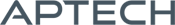# Time Series MT

Time Series MT

Availability: Out of stock

The GAUSS TSMT application module provides a comprehensive suite of tools for MLE and state-space estimation, model diagnostics and forecasting of univariate, multivariate and nonlinear time series models.
Overview

## Time Series MT 3.0

New Times Series MT 3.0   provides for comprehensive treatment of time series models, including model diagnostics, MLE and state-space estimation, and forecasts. Time Series MT also includes tools for managing panel series data and estimating and diagnosing panel series models, including random effects and fixed effects.

Platform: Windows, Mac and Linux.

Requirements: GAUSS/GAUSS Engine 18 or higher.

Features

## Univariate Time-Series Models:

### Conditional mean models:

• Autoregressive moving average (ARMA)
• Seasonal autoregressive moving average (SARMA)
• Autoregressive moving average with exogenous variables (ARMAX)
• Autoregressive integrated moving average (ARIMA)
• Seasonal autoregressive integrated moving average (SARIMA)

### Conditional variance models:

• Generalized autoregressive conditional heteroscedasticity (GARCH)
• GARCH with a unit root (IGARCH)
• GARCH with asymmetrical effects (GJRGARCH)
• GARCH-in-mean (GARCHM)

## Multivariate Time-Series Models:

### Conditional mean models:

• Vector autoregressive moving average (VARMA)
• Vector autoregressive moving average with exogenous variables (VARMAX)
• Seasonal vector autoregressive moving average (SVARMA)
• Seasonal vector autoregressive moving average with exogenous variables (SVARMAX)
• Vector error correction models (VECM)

### Panel Data and other Models:

• Fixed effects and random effects models (TSCS)
• Least squares dummy variable (LSDV)
• Kalman Filter for state-space modeling.

## Nonlinear Time Series Models:

• Switching regression
• Structural break models
• Threshold autoregressive models (TAR)

## Parameter instability tests:

• Chow forecast
• CUSUM Test of Coefficient Equality
• Hansen-Nymblom test
• Rolling Regressions

## Unit Root and Cointegration tests

• Augmented Dickey-Fuller
• Breitung and Das
• Im, Pesaran, and Shin (IPS)
• Johansen’s trace and maximum eigenvalue statistic
• Levin-Lin-Chu (LLC)
• Phillips-Perron
• Zivot and Andrews

## Model Selection and assessment

• Akaike information criterion (AIC)
• Schwartz Bayesian information criterion (BIC)
• Kwiatkowski–Phillips–Schmidt–Shin (KPSS)
• Likelihood ratio statistic (LRS)
• Multivariate Portmanteau statistic
• Wald statistic
• Friedman, Frees and Pesaran tests for cross-sectional independence in panel data models.

Examples

## Nonlinear Time Series Models:

Product Inquiry

2 + 7 = enter the result ex:(3 + 2 = 5)

﻿